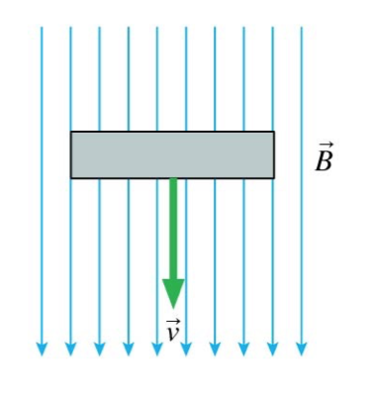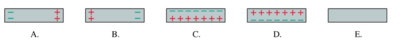# Problem: A metal bar moves through a magnetic field. The induced charges on the bar are

###### FREE Expert Solution

The magnetic force experienced by charges in a moving conducting rod is:

$\overline{){{\mathbf{F}}}_{{\mathbf{B}}}{\mathbf{=}}{\mathbf{q}}{\mathbf{v}}{\mathbf{B}}{\mathbf{s}}{\mathbf{i}}{\mathbf{n}}{\mathbf{\theta }}}$

84% (393 ratings)###### Problem Details

A metal bar moves through a magnetic field. The induced charges on the bar are